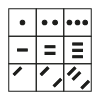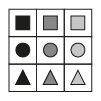# Apply the Pattern to Find the Missing Symbol

In this worksheet, students will find the missing symbol to complete a grid of nine boxes. It will develop their ability to find and follow patterns in shapes and symbols.This content is premium and exclusive to EdPlace subscribers.Key stage:  KS 2

Curriculum topic:   Spatial and Non-Verbal Reasoning

Curriculum subtopic:   Complete the Grid

Difficulty level:#### Worksheet Overview

It’s time to be a puzzle perfecter!

In these questions, we have been given a grid and we need to work out the missing puzzle piece.

The grid is sometimes called a matrix.

Grids can follow a horizontal pattern which goes across, a vertical pattern which goes up or down, or a diagonal pattern.

To find the missing puzzle piece, we first need to think about what the pattern might be.

Here are some example patterns and how they change in the grid:

 Pattern Example Grid Number of shapes in the image increases/decreases.The shading changes.Size of the image increases/decreases.The image rotates.Shapes within the symbol change position.The number of sides of the shapes in the image changes.Take a look at this example:Which of the following images would complete this grid?

a)b)c)d)The pattern in this case is horizontal.

The pattern is that the number of sides of each shape increases by one each time. Also, the centre shape gets slightly larger each time. Therefore the answer is b.

It’s now your turn to find the missing puzzle piece in grids. Remember to decide if it is horizontal, vertical or diagonal, when working out what the pattern is.

Good luck puzzle champ!